Ex 2.2

Chapter 2 Class 9 Polynomials (Term 2)
Serial order wise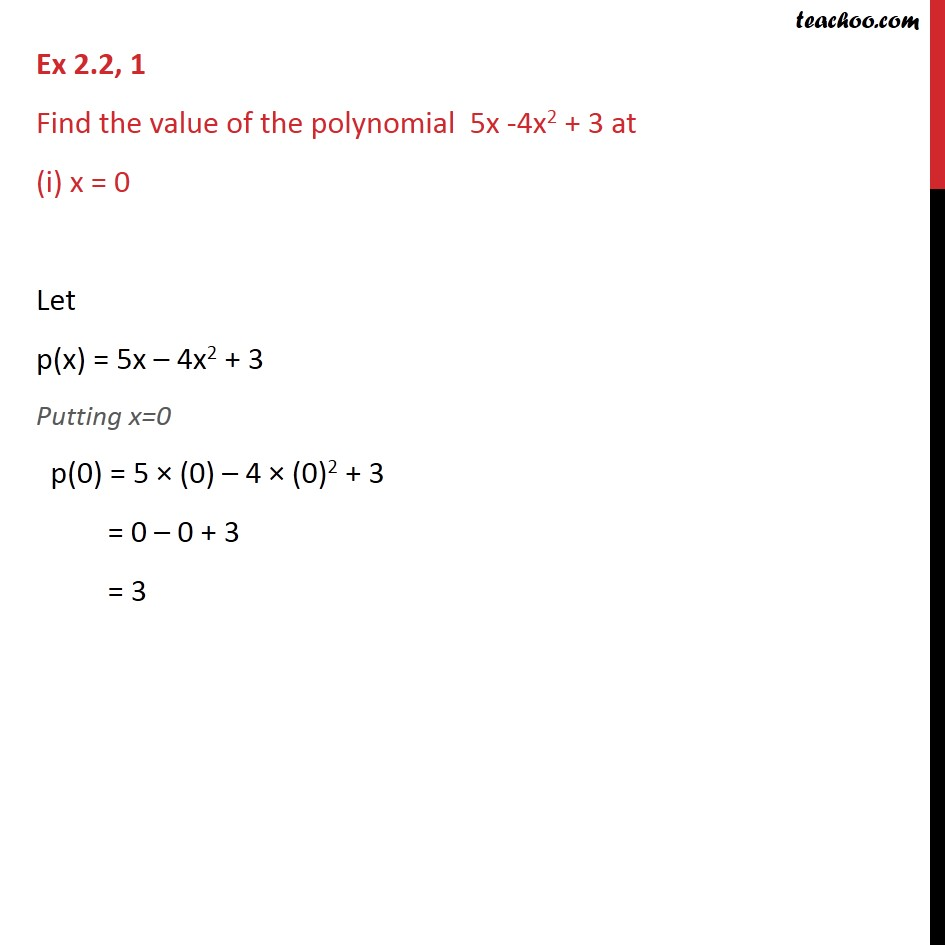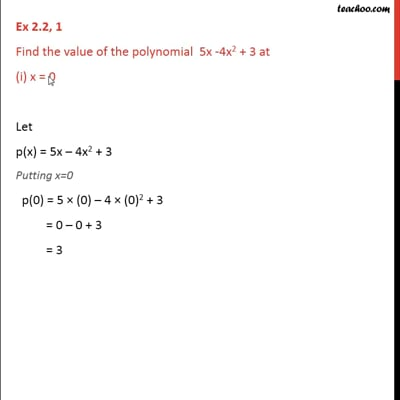This video is only available for Teachoo black users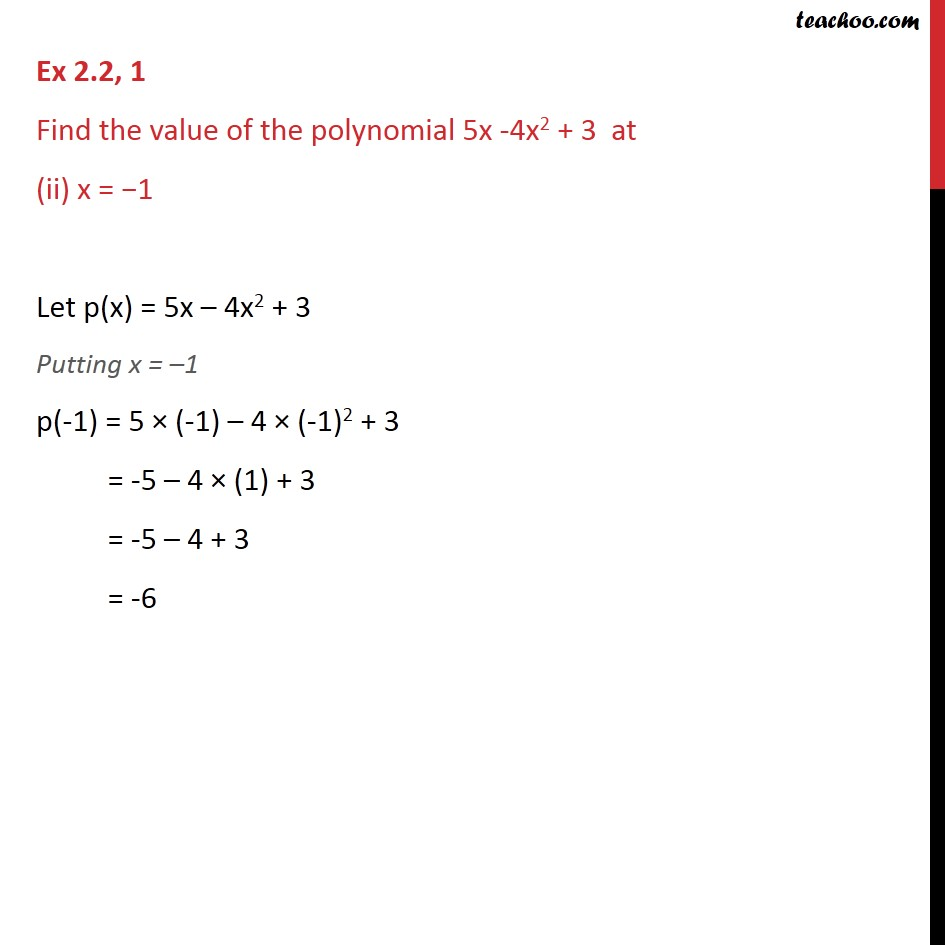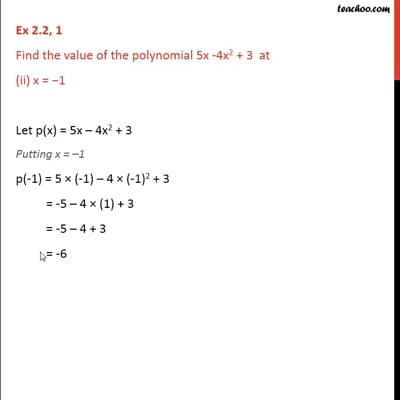This video is only available for Teachoo black users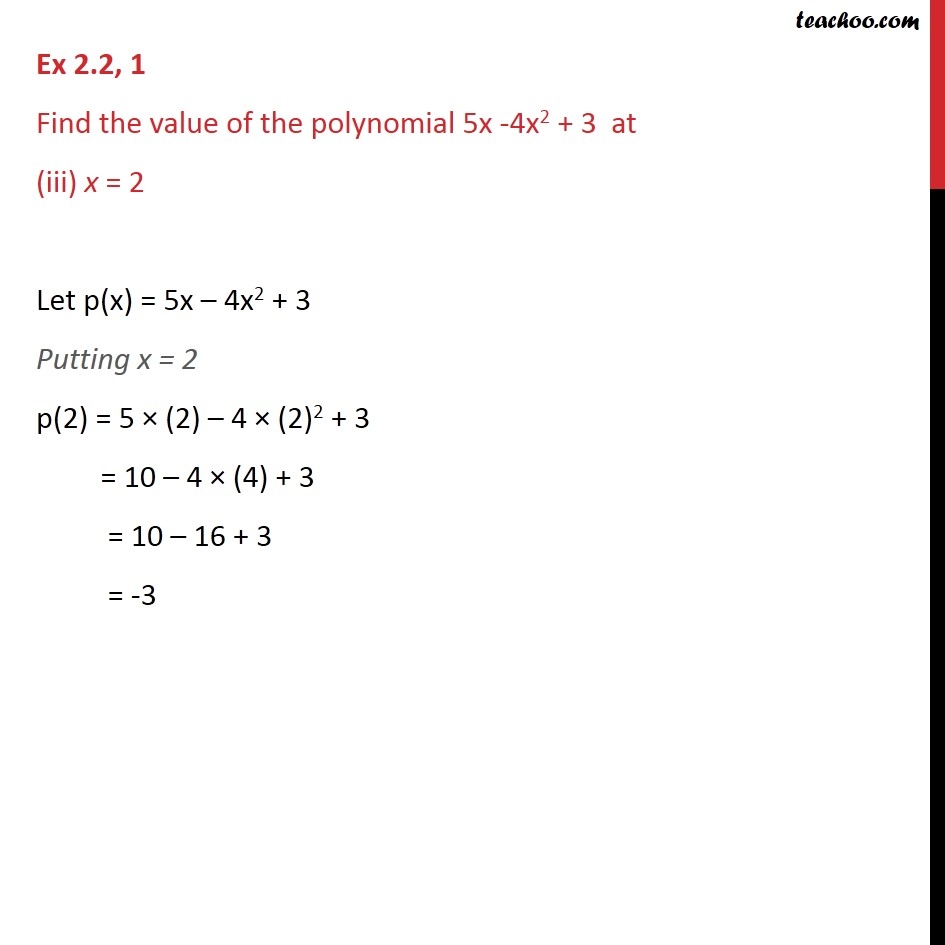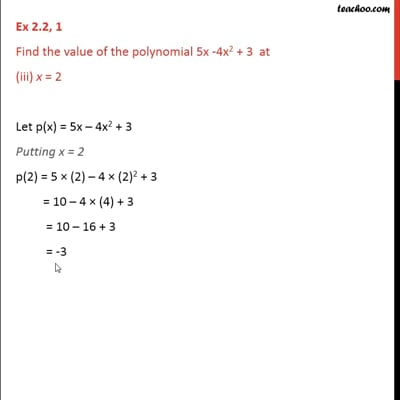This video is only available for Teachoo black users

### Transcript

Ex 2.2, 1 Find the value of the polynomial 5x -4x2 + 3 at (i) x = 0 Let p(x) = 5x – 4x2 + 3 Putting x=0 p(0) = 5 × (0) – 4 × (0)2 + 3 = 0 – 0 + 3 = 3 Ex 2.2, 1 Find the value of the polynomial 5x -4x2 + 3 at (ii) x = −1 Let p(x) = 5x – 4x2 + 3 Putting x = –1 p(-1) = 5 × (-1) – 4 × (-1)2 + 3 = -5 – 4 × (1) + 3 = -5 – 4 + 3 = -6 Ex 2.2, 1 Find the value of the polynomial 5x -4x2 + 3 at (iii) x = 2 Let p(x) = 5x – 4x2 + 3 Putting x = 2 p(2) = 5 × (2) – 4 × (2)2 + 3 = 10 – 4 × (4) + 3 = 10 – 16 + 3 = -3